# RD Sharma Solutions for Class 8 Maths Chapter 10 Direct and Inverse Variations Exercise 10.2

RD Sharma Solutions for Class 8 Maths Exercise 10.2 Chapter 10 Direct and Inverse Variations are provided here. In Exercise 10.2, we shall study problems based on inverse variations (if two quantities are linked in such a way that if one quantity increases, the corresponding quantity decreases and vice-versa, such a variation is called inverse variation). From the exam point of view, to help students prepare for their exams, our experts at BYJU’S have formulated solutions for RD Sharma Solutions in a very lucid and clear manner. Students can download the pdf from the links given below.

## Download the pdf of RD Sharma Solutions for Class 8 Maths Exercise 10.2 Chapter 10 Direct and Inverse Variations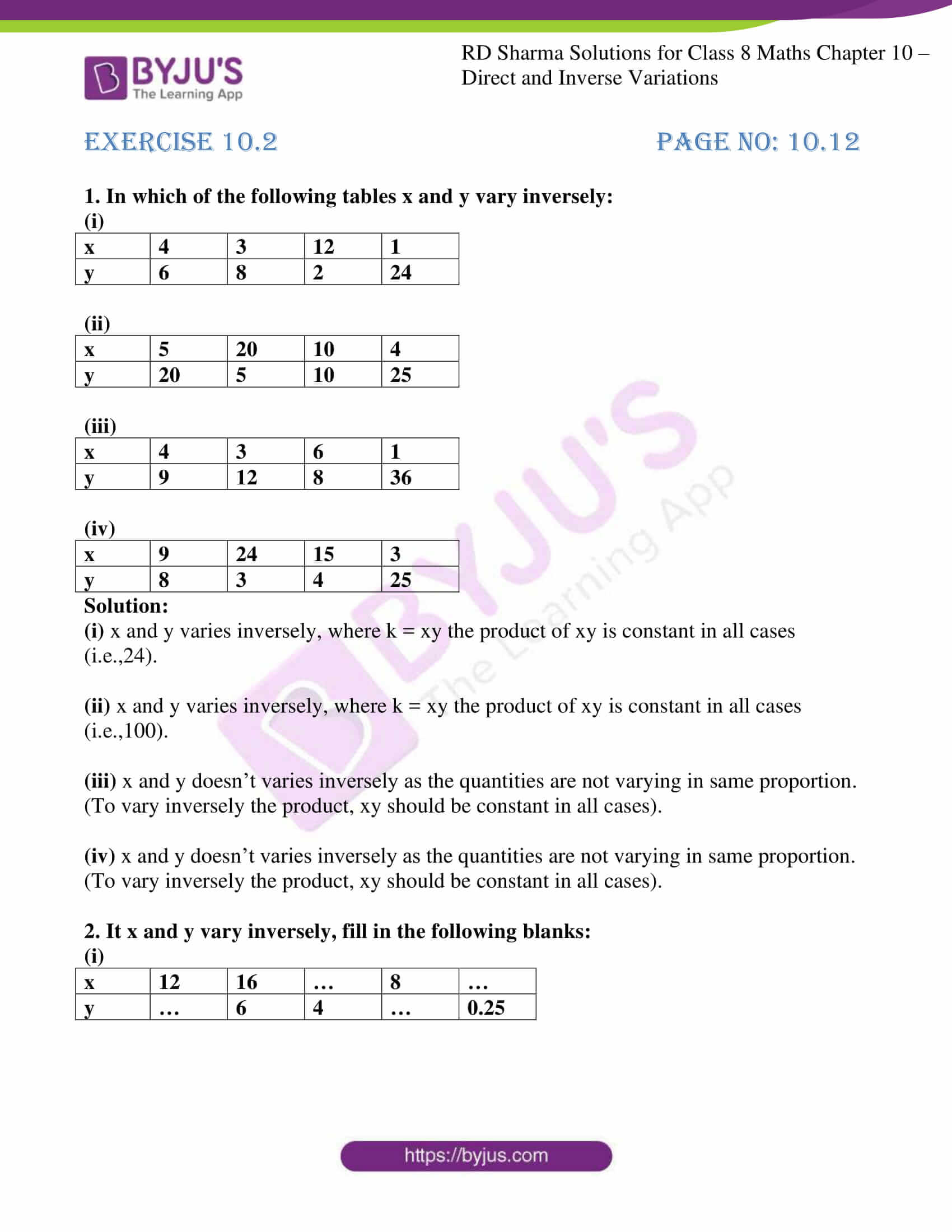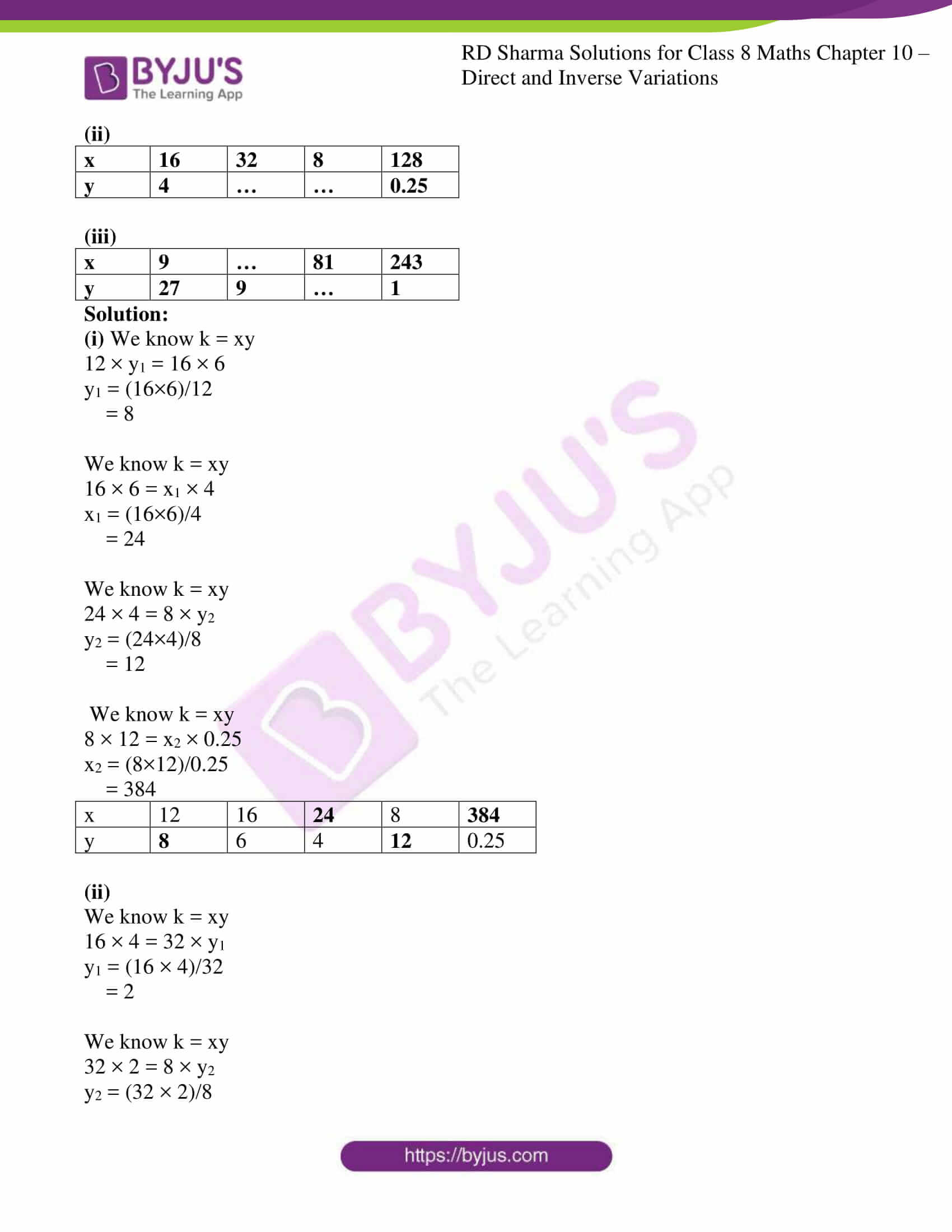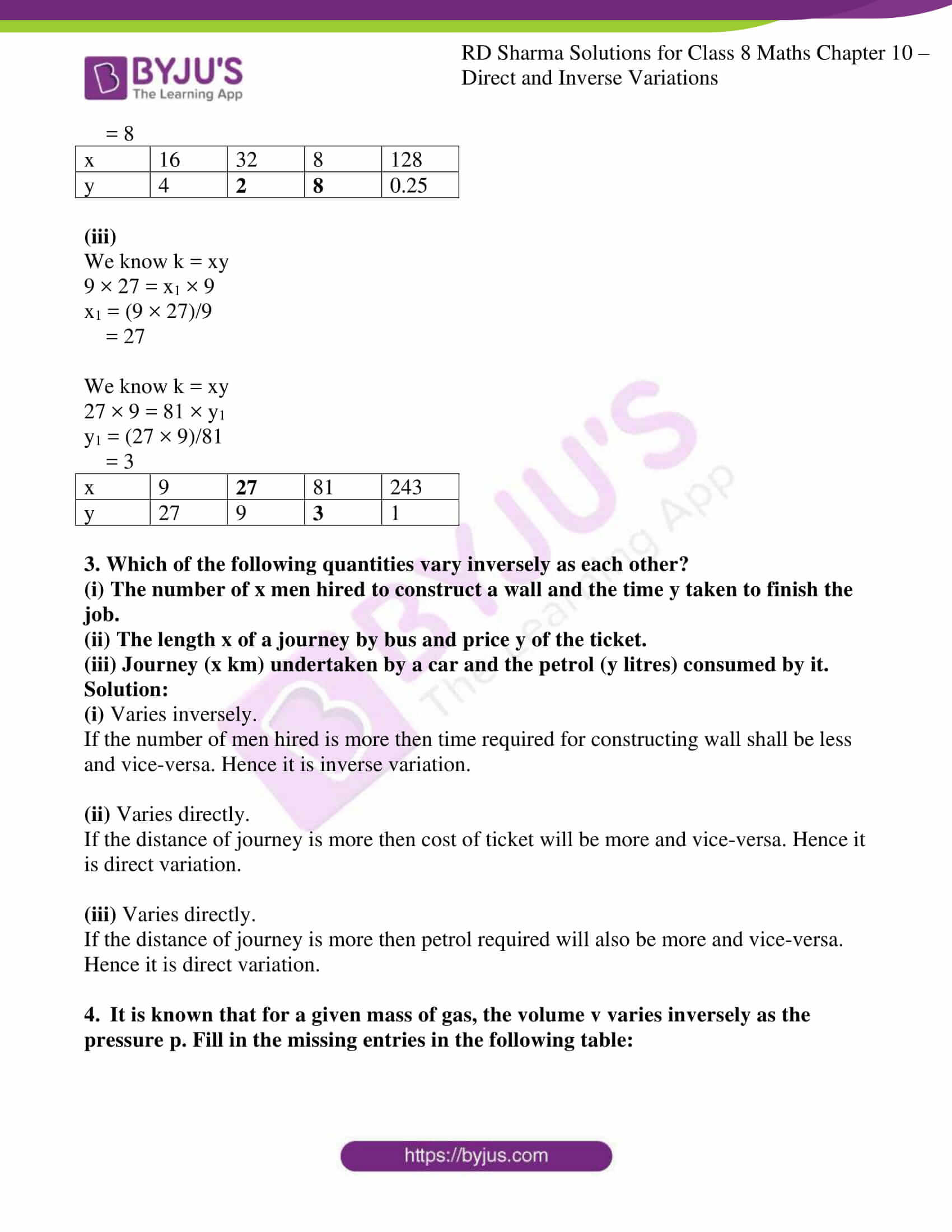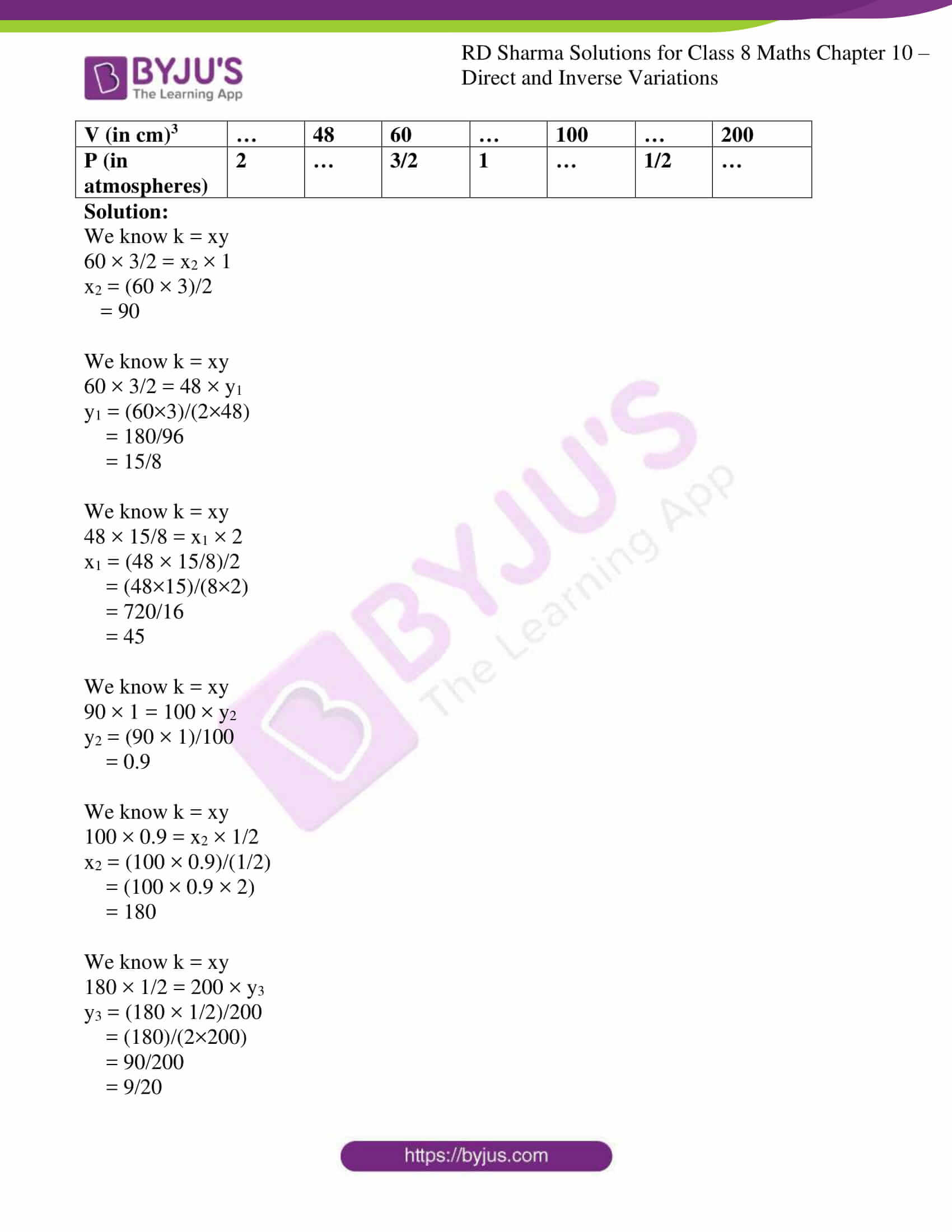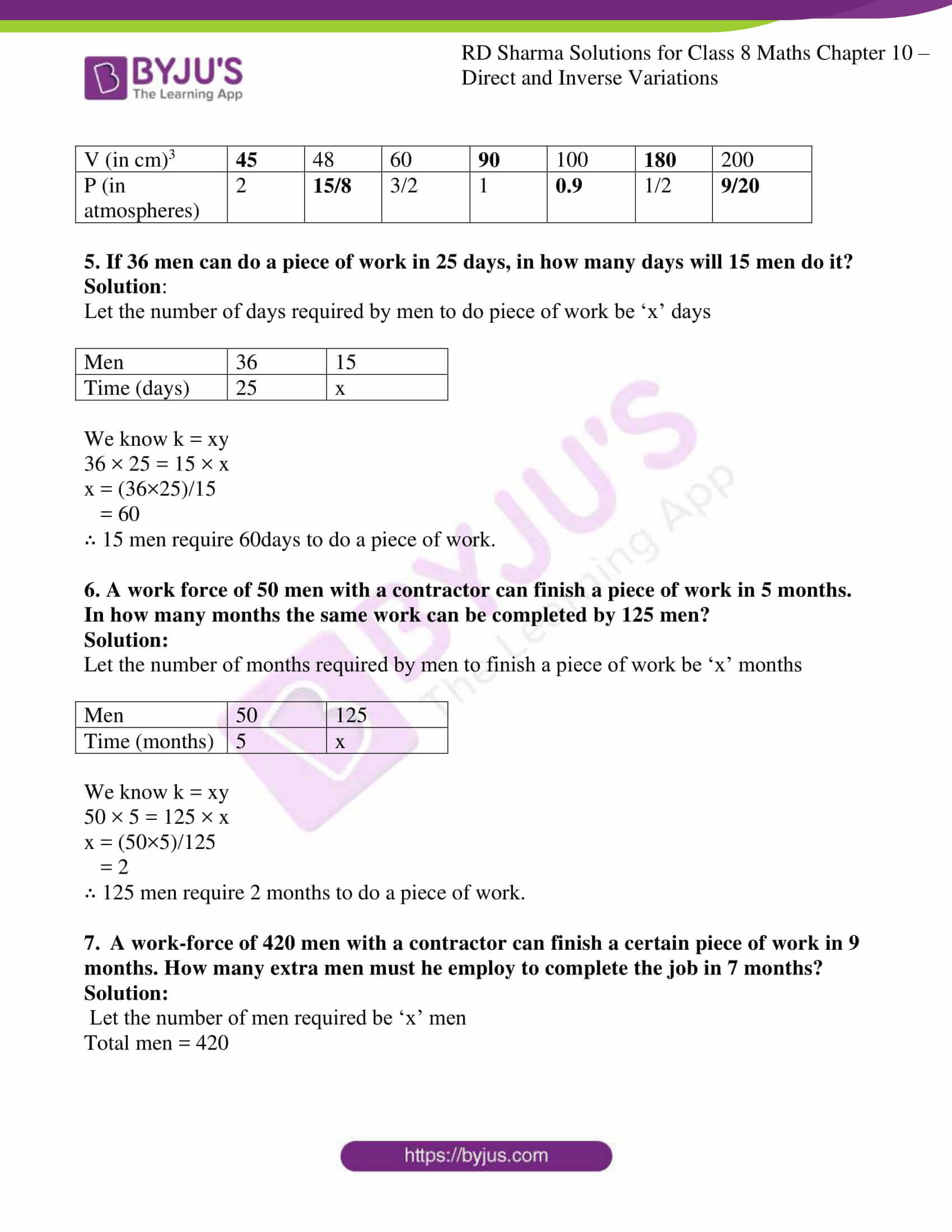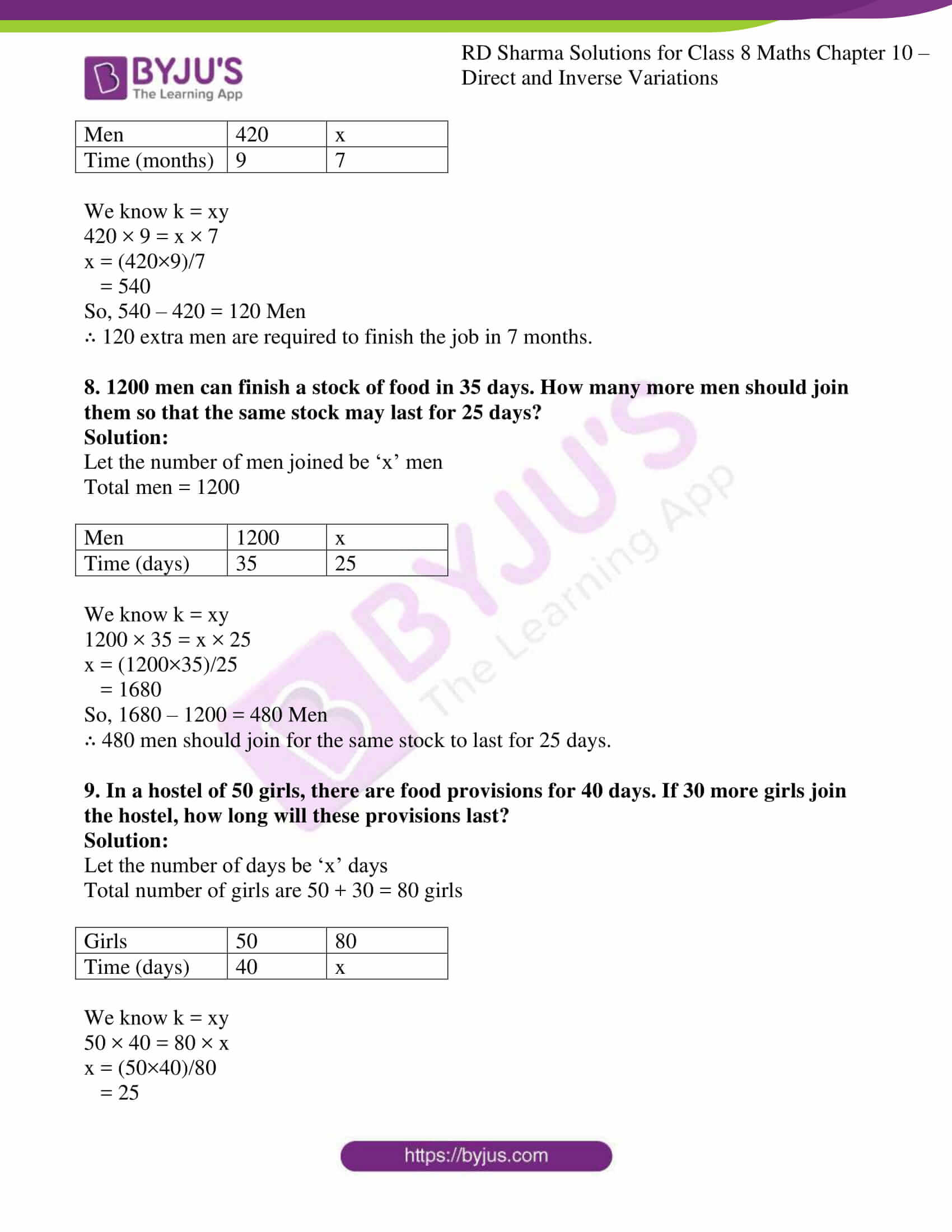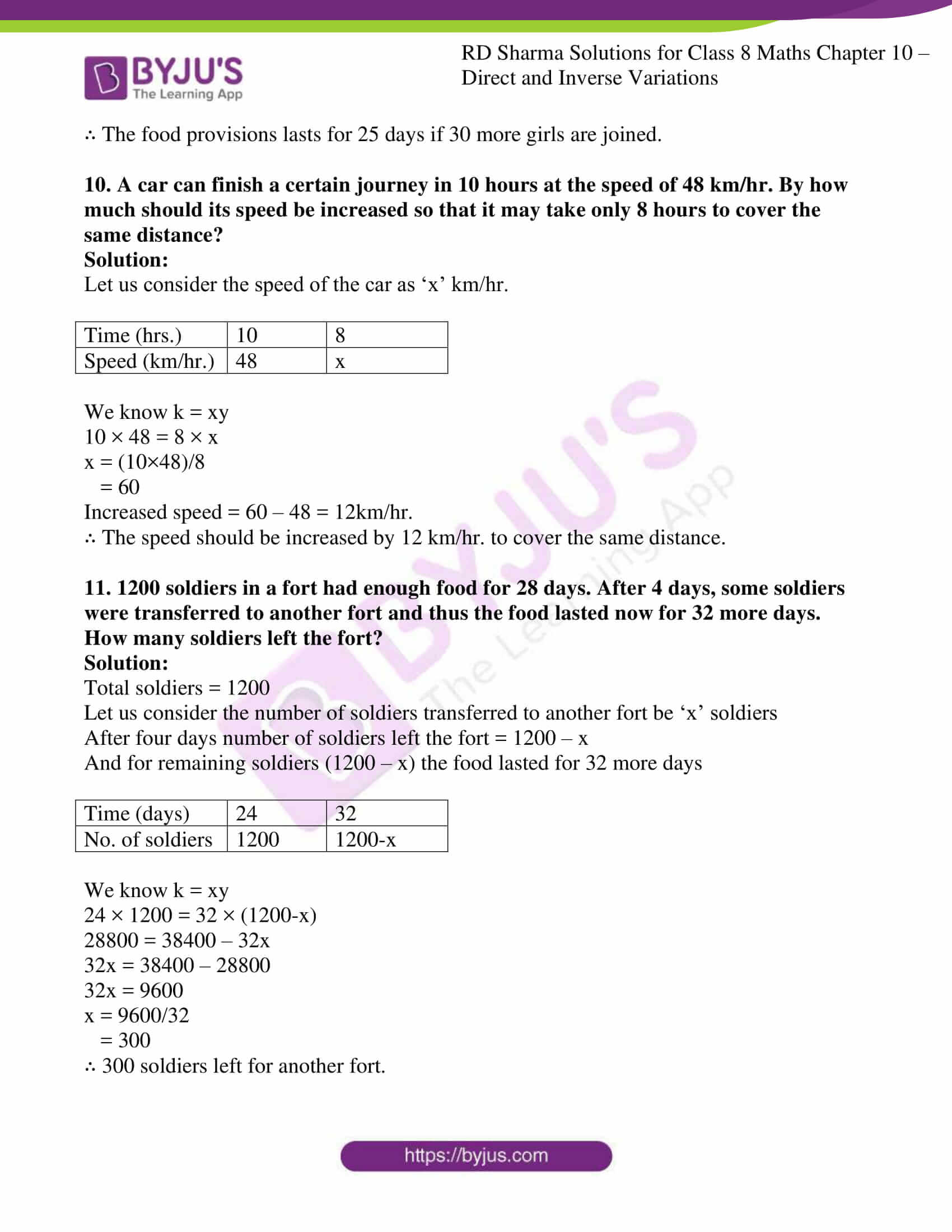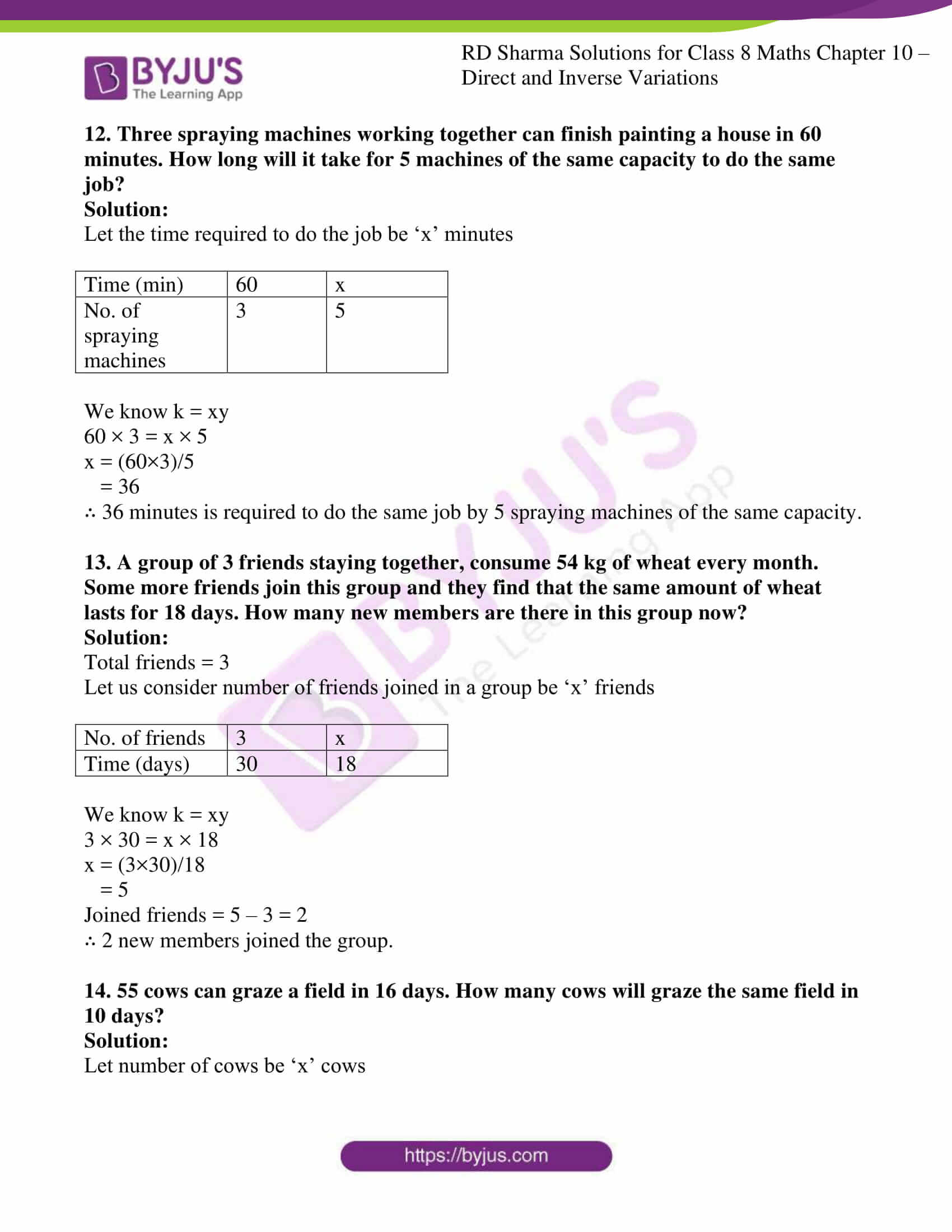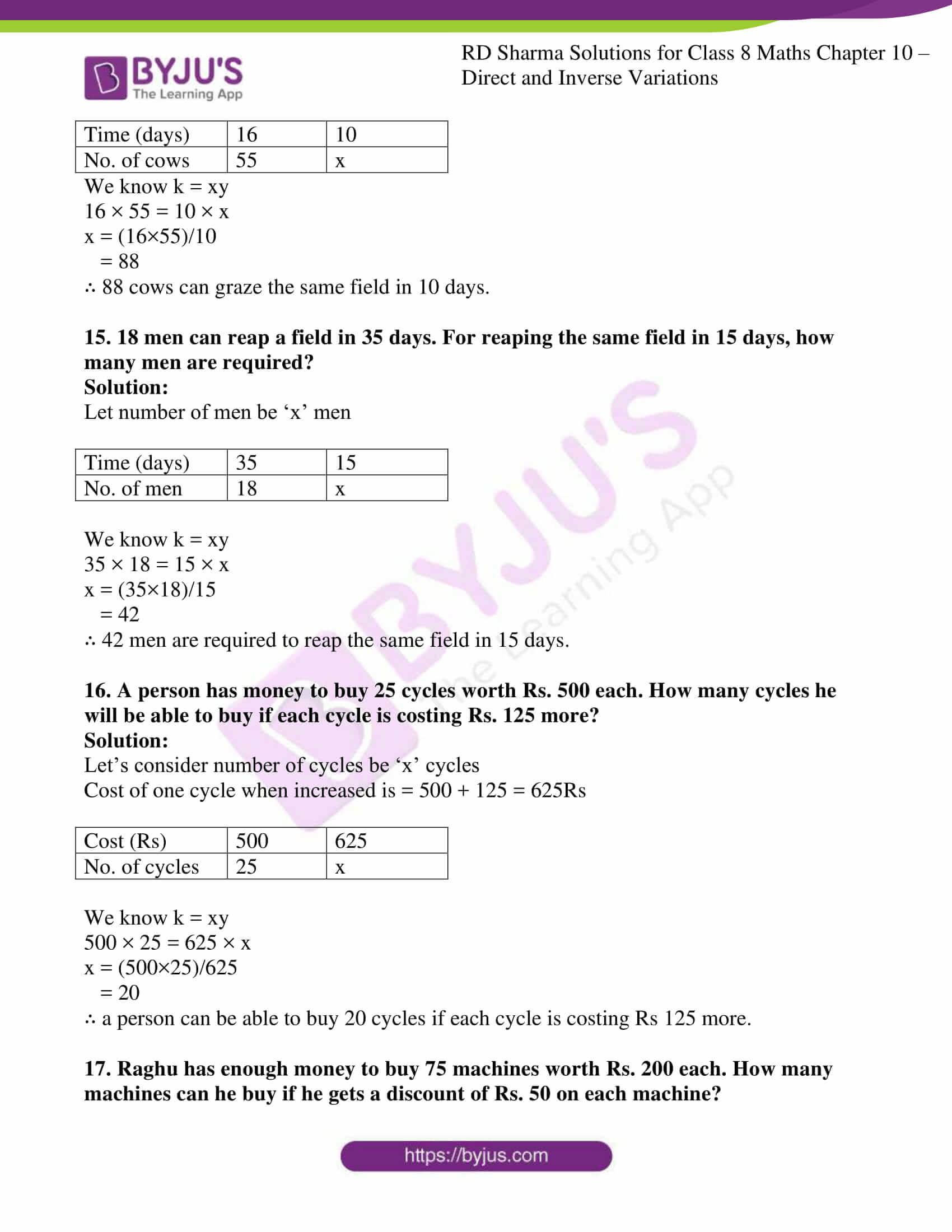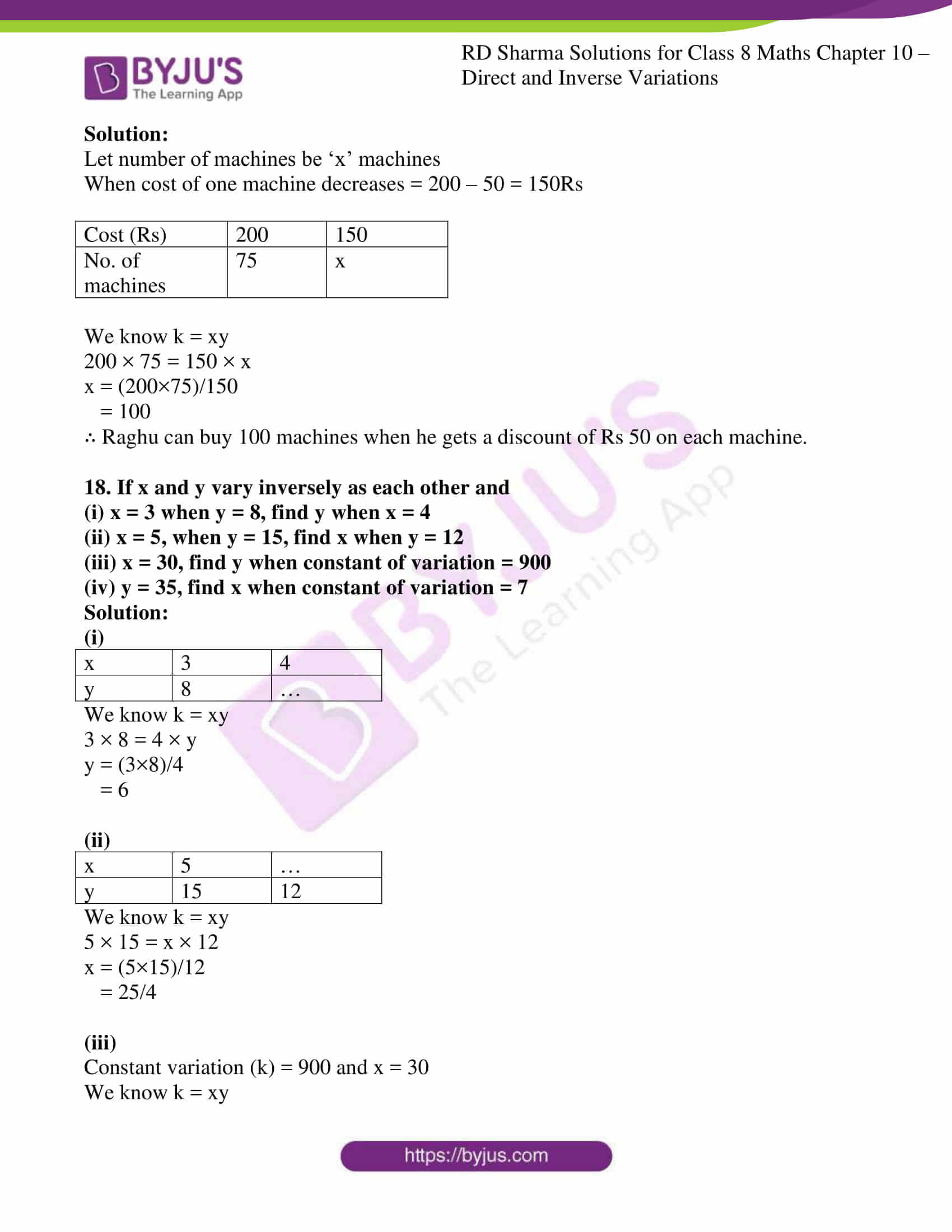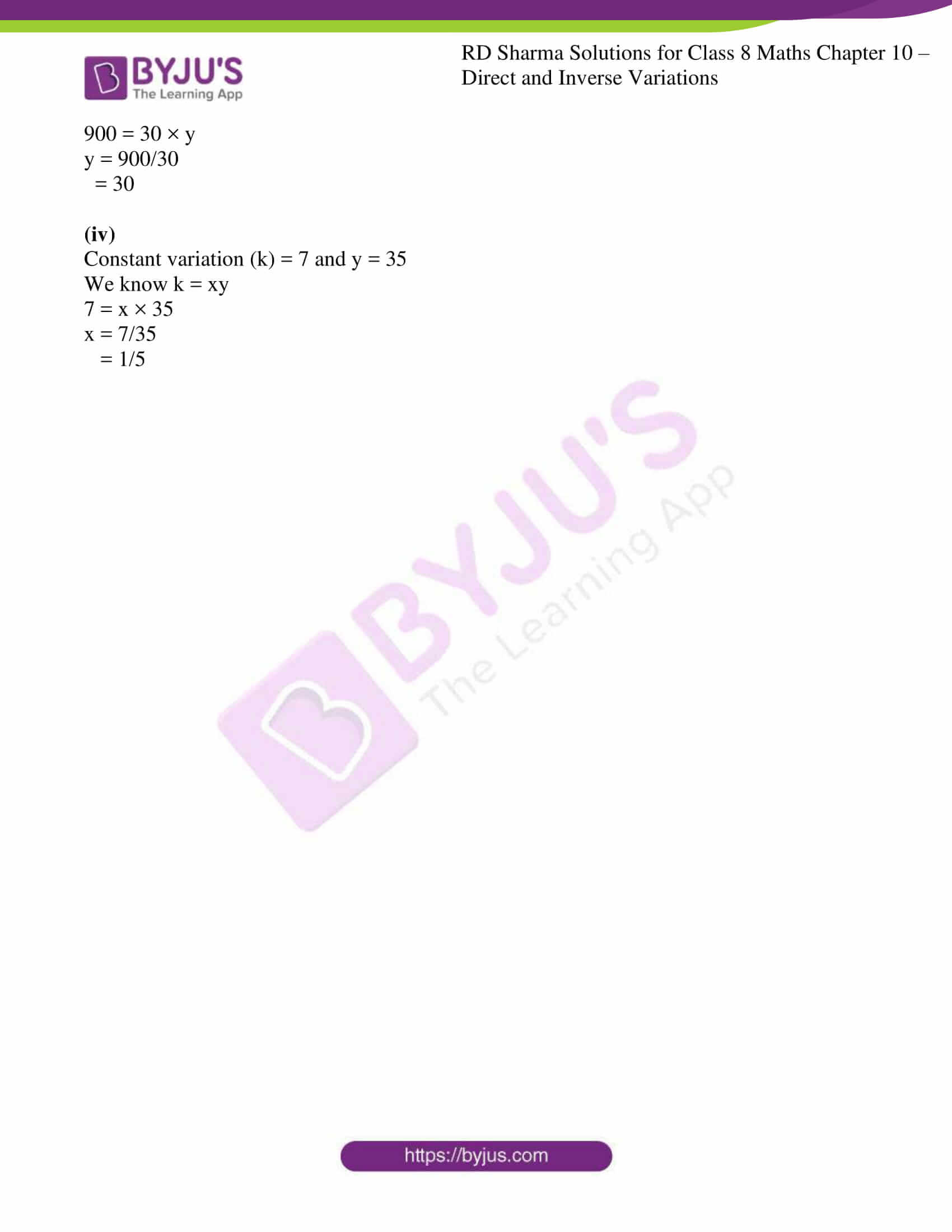### Access Answers to RD Sharma Solutions for Class 8 Maths Exercise 10.2 Chapter 10 Direct and Inverse Variations

1. In which of the following tables x and y vary inversely:

(i)

 x 4 3 12 1 y 6 8 2 24

(ii)

 x 5 20 10 4 y 20 5 10 25

(iii)

 x 4 3 6 1 y 9 12 8 36

(iv)

 x 9 24 15 3 y 8 3 4 25

Solution:

(i) x and y varies inversely, where k = xy the product of xy is constant in all cases (i.e.,24).

(ii) x and y varies inversely, where k = xy the product of xy is constant in all cases (i.e.,100).

(iii) x and y doesn’t varies inversely as the quantities are not varying in same proportion.

(To vary inversely the product, xy should be constant in all cases).

(iv) x and y doesn’t varies inversely as the quantities are not varying in same proportion.

(To vary inversely the product, xy should be constant in all cases).

2. It x and y vary inversely, fill in the following blanks:

(i)

 x 12 16 … 8 … y … 6 4 … 0.25

(ii)

 x 16 32 8 128 y 4 … … 0.25

(iii)

 x 9 … 81 243 y 27 9 … 1

Solution:

(i) We know k = xy

12 × y1 = 16 × 6

y1 = (16×6)/12

= 8

We know k = xy

16 × 6 = x1 × 4

x1 = (16×6)/4

= 24

We know k = xy

24 × 4 = 8 × y2

y2 = (24×4)/8

= 12

We know k = xy

8 × 12 = x2 × 0.25

x2 = (8×12)/0.25

= 384

 x 12 16 24 8 384 y 8 6 4 12 0.25

(ii)

We know k = xy

16 × 4 = 32 × y1

y1 = (16 × 4)/32

= 2

We know k = xy

32 × 2 = 8 × y2

y2 = (32 × 2)/8

= 8

 x 16 32 8 128 y 4 2 8 0.25

(iii)

We know k = xy

9 × 27 = x1 × 9

x1 = (9 × 27)/9

= 27

We know k = xy

27 × 9 = 81 × y1

y1 = (27 × 9)/81

= 3

 x 9 27 81 243 y 27 9 3 1

3. Which of the following quantities vary inversely as each other?
(i) The number of x men hired to construct a wall and the time y taken to finish the job.
(ii) The length x of a journey by bus and price y of the ticket.
(iii) Journey (x km) undertaken by a car and the petrol (y litres) consumed by it.

Solution:

(i) Varies inversely.

If the number of men hired is more then time required for constructing wall shall be less and vice-versa. Hence it is inverse variation.

(ii) Varies directly.

If the distance of journey is more then cost of ticket will be more and vice-versa. Hence it is direct variation.

(iii) Varies directly.

If the distance of journey is more then petrol required will also be more and vice-versa. Hence it is direct variation.

4. It is known that for a given mass of gas, the volume v varies inversely as the pressure p. Fill in the missing entries in the following table:

 V (in cm)3 … 48 60 … 100 … 200 P (in atmospheres) 2 … 3/2 1 … 1/2 …

Solution:

We know k = xy

60 × 3/2 = x2 × 1

x2 = (60 × 3)/2

= 90

We know k = xy

60 × 3/2 = 48 × y1

y1 = (60×3)/(2×48)

= 180/96

= 15/8

We know k = xy

48 × 15/8 = x1 × 2

x1 = (48 × 15/8)/2

= (48×15)/(8×2)

= 720/16

= 45

We know k = xy

90 × 1 = 100 × y2

y2 = (90 × 1)/100

= 0.9

We know k = xy

100 × 0.9 = x2 × 1/2

x2 = (100 × 0.9)/(1/2)

= (100 × 0.9 × 2)

= 180

We know k = xy

180 × 1/2 = 200 × y3

y3 = (180 × 1/2)/200

= (180)/(2×200)

= 90/200

= 9/20

 V (in cm)3 45 48 60 90 100 180 200 P (in atmospheres) 2 15/8 3/2 1 0.9 1/2 9/20

5. If 36 men can do a piece of work in 25 days, in how many days will 15 men do it?

Solution:

Let the number of days required by men to do piece of work be ‘x’ days

 Men 36 15 Time (days) 25 x

We know k = xy

36 × 25 = 15 × x

x = (36×25)/15

= 60

∴ 15 men require 60days to do a piece of work.

6. A work force of 50 men with a contractor can finish a piece of work in 5 months. In how many months the same work can be completed by 125 men?

Solution:

Let the number of months required by men to finish a piece of work be ‘x’ months

 Men 50 125 Time (months) 5 x

We know k = xy

50 × 5 = 125 × x

x = (50×5)/125

= 2

∴ 125 men require 2 months to do a piece of work.

7. A work-force of 420 men with a contractor can finish a certain piece of work in 9 months. How many extra men must he employ to complete the job in 7 months?

Solution:

Let the number of men required be ‘x’ men

Total men = 420

 Men 420 x Time (months) 9 7

We know k = xy

420 × 9 = x × 7

x = (420×9)/7

= 540

So, 540 – 420 = 120 Men

∴ 120 extra men are required to finish the job in 7 months.

8. 1200 men can finish a stock of food in 35 days. How many more men should join them so that the same stock may last for 25 days?

Solution:

Let the number of men joined be ‘x’ men

Total men = 1200

 Men 1200 x Time (days) 35 25

We know k = xy

1200 × 35 = x × 25

x = (1200×35)/25

= 1680

So, 1680 – 1200 = 480 Men

∴ 480 men should join for the same stock to last for 25 days.

9. In a hostel of 50 girls, there are food provisions for 40 days. If 30 more girls join the hostel, how long will these provisions last?

Solution:

Let the number of days be ‘x’ days

Total number of girls are 50 + 30 = 80 girls

 Girls 50 80 Time (days) 40 x

We know k = xy

50 × 40 = 80 × x

x = (50×40)/80

= 25

∴ The food provisions lasts for 25 days if 30 more girls are joined.

10. A car can finish a certain journey in 10 hours at the speed of 48 km/hr. By how much should its speed be increased so that it may take only 8 hours to cover the same distance?

Solution:

Let us consider the speed of the car as ‘x’ km/hr.

 Time (hrs.) 10 8 Speed (km/hr.) 48 x

We know k = xy

10 × 48 = 8 × x

x = (10×48)/8

= 60

Increased speed = 60 – 48 = 12km/hr.

∴ The speed should be increased by 12 km/hr. to cover the same distance.

11. 1200 soldiers in a fort had enough food for 28 days. After 4 days, some soldiers were transferred to another fort and thus the food lasted now for 32 more days. How many soldiers left the fort?

Solution:

Total soldiers = 1200

Let us consider the number of soldiers transferred to another fort be ‘x’ soldiers

After four days number of soldiers left the fort = 1200 – x

And for remaining soldiers (1200 – x) the food lasted for 32 more days

 Time (days) 24 32 No. of soldiers 1200 1200-x

We know k = xy

24 × 1200 = 32 × (1200-x)

28800 = 38400 – 32x

32x = 38400 – 28800

32x = 9600

x = 9600/32

= 300

∴ 300 soldiers left for another fort.

12. Three spraying machines working together can finish painting a house in 60 minutes. How long will it take for 5 machines of the same capacity to do the same job?

Solution:

Let the time required to do the job be ‘x’ minutes

 Time (min) 60 x No. of spraying machines 3 5

We know k = xy

60 × 3 = x × 5

x = (60×3)/5

= 36

∴ 36 minutes is required to do the same job by 5 spraying machines of the same capacity.

13. A group of 3 friends staying together, consume 54 kg of wheat every month. Some more friends join this group and they find that the same amount of wheat lasts for 18 days. How many new members are there in this group now?

Solution:

Total friends = 3

Let us consider number of friends joined in a group be ‘x’ friends

 No. of friends 3 x Time (days) 30 18

We know k = xy

3 × 30 = x × 18

x = (3×30)/18

= 5

Joined friends = 5 – 3 = 2

∴ 2 new members joined the group.

14. 55 cows can graze a field in 16 days. How many cows will graze the same field in 10 days?

Solution:

Let number of cows be ‘x’ cows

 Time (days) 16 10 No. of cows 55 x

We know k = xy

16 × 55 = 10 × x

x = (16×55)/10

= 88

∴ 88 cows can graze the same field in 10 days.

15. 18 men can reap a field in 35 days. For reaping the same field in 15 days, how many men are required?

Solution:

Let number of men be ‘x’ men

 Time (days) 35 15 No. of men 18 x

We know k = xy

35 × 18 = 15 × x

x = (35×18)/15

= 42

∴ 42 men are required to reap the same field in 15 days.

16. A person has money to buy 25 cycles worth Rs. 500 each. How many cycles he will be able to buy if each cycle is costing Rs. 125 more?

Solution:

Let’s consider number of cycles be ‘x’ cycles

Cost of one cycle when increased is = 500 + 125 = 625Rs

 Cost (Rs) 500 625 No. of cycles 25 x

We know k = xy

500 × 25 = 625 × x

x = (500×25)/625

= 20

∴ a person can be able to buy 20 cycles if each cycle is costing Rs 125 more.

17. Raghu has enough money to buy 75 machines worth Rs. 200 each. How many machines can he buy if he gets a discount of Rs. 50 on each machine?

Solution:

Let number of machines be ‘x’ machines

When cost of one machine decreases = 200 – 50 = 150Rs

 Cost (Rs) 200 150 No. of machines 75 x

We know k = xy

200 × 75 = 150 × x

x = (200×75)/150

= 100

∴ Raghu can buy 100 machines when he gets a discount of Rs 50 on each machine.

18. If x and y vary inversely as each other and
(i) x = 3 when y = 8, find y when x = 4
(ii) x = 5, when y = 15, find x when y = 12
(iii) x = 30, find y when constant of variation = 900
(iv) y = 35, find x when constant of variation = 7

Solution:

(i)

 x 3 4 y 8 …

We know k = xy

3 × 8 = 4 × y

y = (3×8)/4

= 6

(ii)

 x 5 … y 15 12

We know k = xy

5 × 15 = x × 12

x = (5×15)/12

= 25/4

(iii)

Constant variation (k) = 900 and x = 30

We know k = xy

900 = 30 × y

y = 900/30

= 30

(iv)

Constant variation (k) = 7 and y = 35

We know k = xy

7 = x × 35

x = 7/35

= 1/5